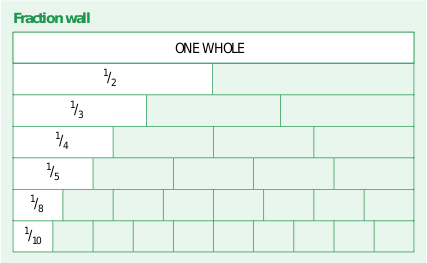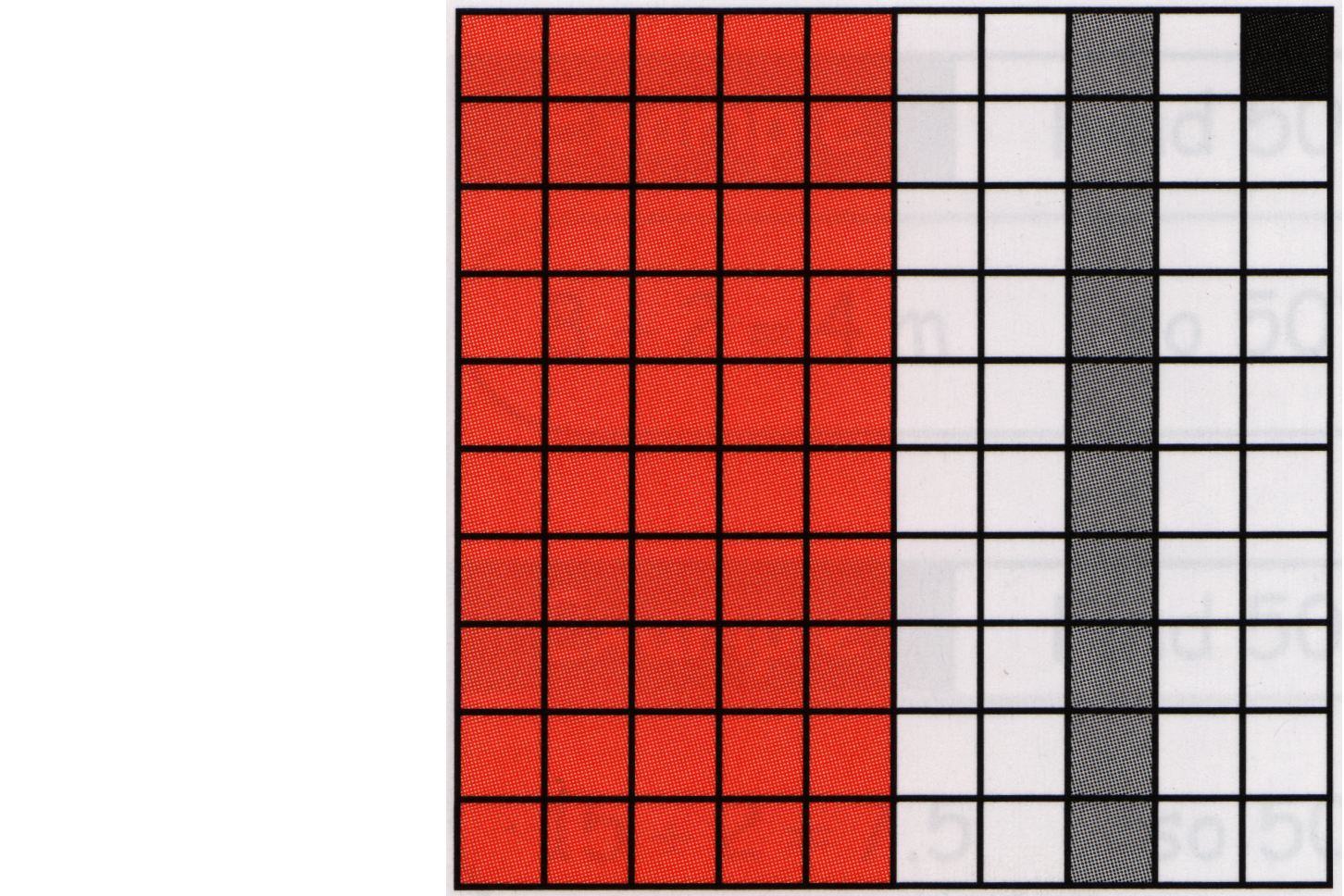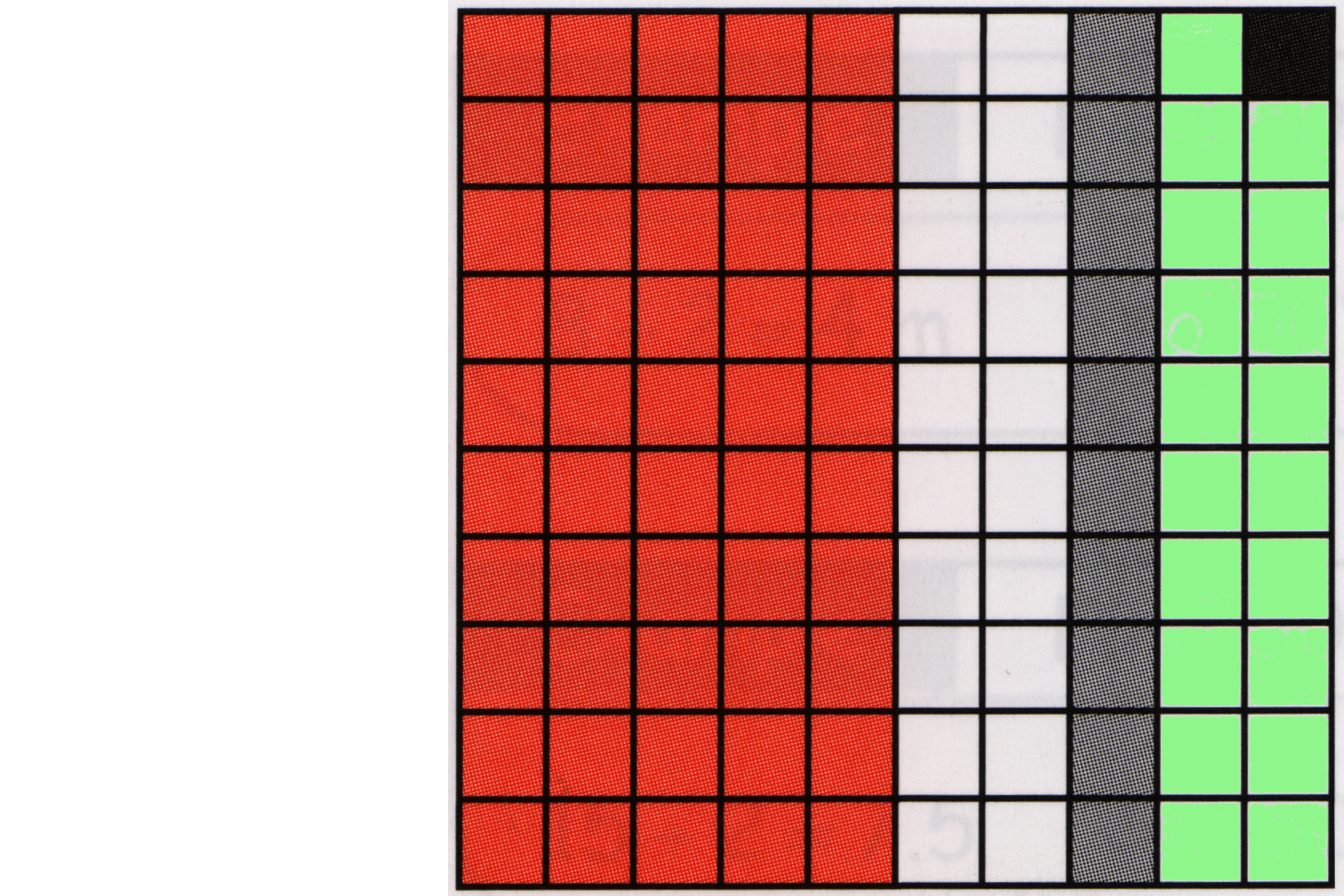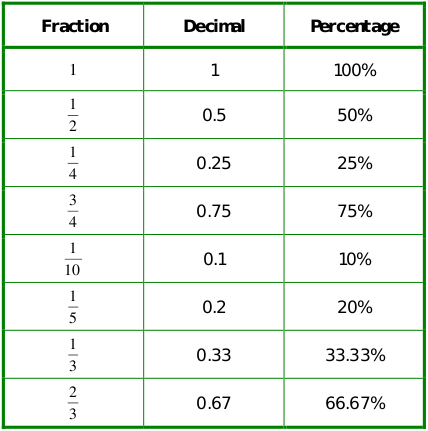## List of Slides:

Nurse's Maths: Blackberry: Project Marks: A trainee nurse has to complete and hand in an important piece of academic work at university. There are two sections which both need improvement; an essay and a case study. But there is only enough time to work on one. The essay part needs more research, a review of the ideas discussed, and a careful rewrite of the summary. On the other hand, the case study needs a lot of data analysis, charts and tables, printing and layout. Looking at the marking scheme, the essay could probably be worth about 20% more if it was worked on. The case study might earn about 25% percent more with the extra charts and data analysis. The project will be marked out of a total of 100 marks. The essay will be marked out of 60, the case study out of 40. The trainee nurse needs the best grade possible for this work. Ignoring any personal preferences, where should the extra effort be put in? To give our trainee nurse the best advice: 1) Work out how many extra marks could be earned by improving the essay (marked out of 60). 2) Work out how many extra marks could be earned by improving the case study (marked out of 40). 45% of 40 is 4x10% + 1/2 of 10% = 16 marks + 2 marks = 18 marks 35% of 60 is 3x10% + 1/2 of 10% = 18 marks + 3 marks = 21 marks Coding Improvements: Cosmaetic - set nav buttons consistently [not broken] Efficiency - make nav work direct from edit+save+refresh browser Problem is Kompozer deletes nav code, which introduces an extra reload and save n an extra application. Solotion option 1) add a document write sequence in body to repair (or fully insert) nav button page code. Solution option 2) Add object code to create fresh ToC from doc headings, inserting matching nav anchors

## Splash: Percentage## A Very Popular Idea!

From wage increases to...

## Some Benefits of Knowing how to Calculate %

% interest rates can affect your weekly or monthly payments. Mistakes can take a long time to pay off.

% reductions can affect where you shop and what you buy.

## What Is A percentage?

percentage:
a fraction expressed as
the number of parts per hundred

## Parts of A Whole:

Unit fractions...## Parts of A Hundred:## The Green Bit?## Equivalents## Widely-Used Shorcut with Money:

Because there are also 100 pence in a pound,
a percentage change applied to a pound
gives a result in pence.

Pounds x Percentage = Pence

## How It Works:

�1.0 x 45% = pence

(1.0 x 100) x 45/100 = Pence

The percentage is saying "divide by 100"
Pounds to pence means "multiply by 100"
Multiply and divide are opposites, so cancel each other out

## REVIEW: What have we achieved?

1) We can visualise a percentage mathematically.
(2D Grid or fraction)
2) We know the % sign implies "divided by 100"
3) Percentages can compare proportions of a whole
4) Because pence are already (pounds x 100),
percent values work on pounds to give pence

## END

No More Slides Here.

:
.

Thank you.